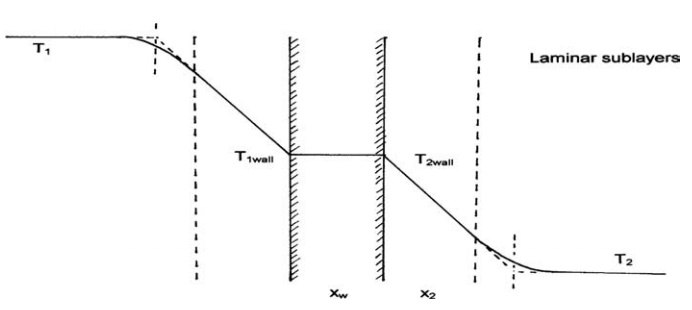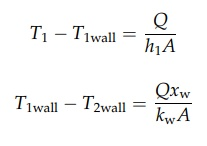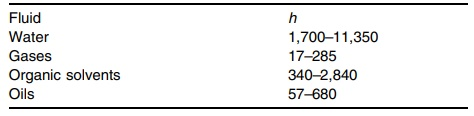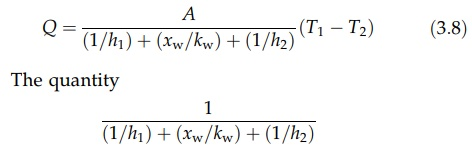# Heat Exchange Between a Fluid and a Solid Boundary

| Home | | Pharmaceutical Technology |

## Chapter: Pharmaceutical Engineering: Heat Transfer

Conduction and convection contribute to the transfer of heat from a fluid to a boundary.

HEAT EXCHANGE BETWEEN A FLUID AND A SOLID BOUNDARY

Conduction and convection contribute to the transfer of heat from a fluid to a boundary. The distribution of temperatures at a plane barrier separating two fluids is shown in Figure 3.2. If the fluids are in turbulent motion, temperature gradients are confined to a relatively narrow region adjacent to the wall. Outside this region, turbulent mixing, the mechanism of which is explained in chapter 2, is very effective in the transfer of heat. Temperature gradients are quickly destroyed, and equalization of values T1 and T2 occurs. Within the region, there exists a laminar sublayer across which heat is transferred by conduction only. The thermal conductivity of most fluids is small, as shown in Table 3.1. TheFIGURE 3.2 Heat transfer between fluids.

temperature gradients produced by a given heat flow are correspondingly high. Outside the laminar layer, eddies contribute to the transfer of heat by moving fluid from the turbulent bulk to the edge of the sublayer, where heat can be lost or gained, and by corresponding movements in the opposite direction. The temperature gradients in this region, where both convection and conduction contribute to heat transfer, are smaller than that in the sublayer.

The major resistance to heat flow resides in the laminar sublayer. Its thickness is, therefore, of critical importance in determining the rate of heat transfer from the fluid to the boundary. It depends on the physical properties of the fluid, the flow conditions, and the nature of the surface. Increase in flow velocity, for example, decreases the thickness of the layer and, therefore, its resistance to heat flow. The interaction of these variables is exceedingly complex.

A film transmitting heat only by conduction may be postulated to evaluate the rate of heat transfer at a boundary. This fictitious film presents the same resistance to heat transfer as the complex turbulent and laminar regions near the wall. If, on the hot side of the wall, the fictitious layer had a thickness x1, the equation of heat transfer to the wall would bewhere k is the thermal conductivity of the fluid. A similar equation will apply to heat transfer at the cold side of the wall. The thickness of the layer is determined by the same factors that control the extent of the laminar sublayer. In general, it is not known and the equation above may be rewritten as

Q = h1A(T1 - T1wall)                              (3:7)

where h1 is the heat transfer coefficient for the film under discussion. It corre-sponds to the ratio k/x1 and has units J/m2·sec·K. This is a convenient, numerical expression of the flow of heat by conduction and convection at a boundary. Typical values of heat transfer or film coefficients are given in Table 3.2. The approximate evaluation of these coefficients is discussed in the next section.

The ratio of the temperature difference and the total thermal resistance determines the rate of heat transfer across the three layers of Figure 3.2. Using the film coefficient h2 to characterize heat transfer from the barrier to the colder fluid,TABLE 3.2 Film Coefficient, h, for Various Fluids (J/m2 sec K)where kw is the thermal conductivity of the wall.Addition and rearrangement of these equations giveis called the overall heat transfer coefficient, U. A general expression of the rate of heat transfer then becomes

Q = UAΔT                       (3:9)